# Meaning of Perimeter | Perimeter of Triangle, Square and Rectangular Shapes | Skip Count 861 – 880 Primary 3 (Basic 3) Term 3 Week 3 Mathematics

### MATHEMATICS

THIRD TERM

WEEK 4

PRIMARY 3

THEME – PRIMARY MEASURES

PREVIOUS LESSON – Length – Non Standard and Non Standard Measurement | Measuring in Centimetres and Metres | Real Life Application | Counting 841 – 860 Primary 3 (Basic 3) Term 3 Week 2 Mathematics

### LEARNING AREA

1. Introduction

2. Perimeter of a Triangle

3. Perimeter of a Square

4. Perimeter of a Rectangle

5. Lesson Evaluation and Weekly Assessment (Test)

### PERFORMANCE OBJECTIVES

By the end of the lesson, most pupils should have attained the following objectives –

1. define perimeter.

2. find perimeters of regular figures in metres and centimeters by measurement.

3. identify perimeter of regular shapes in their environments.

4. realize that perimeter is the total distance round a particular shape.

### RATIONALE

Perimeter is the distance round a particular shape. The perimeter of a rectangle or square is to add all the four sides. The concept of perimeter will enable the pupils to understand and appreciate the distance around a particular shape or object.

### ENTRY BEHAVIOUR

The pupils can add four (4) numbers together.

### INSTRUCTIONAL MATERIALS

The teacher will teach the lesson with the aid of cut-outs of squares, rectangle, triangles and teacher’s table.

### METHOD OF TEACHING

Choose a suitable and appropriate methods for the lessons.

Note – Irrespective of choosing methods of teaching, always introduce an activities that will arouse pupil’s interest or lead them to the lessons.

### REFERENCE MATERIALS

1. Scheme of Work

2. 9 – Years Basic Education Curriculum

3. Course Book

4. All Relevant Material

5. Online Information

### LESSON 1 – SKIP COUNT 861 – 880

ACTIVITY 1 – COUNT 861 – 880

861  862  863  864  865  846  867  868  869  870

871  872  873  874  875  876  877  878  879  880

ACTIVITY 2 – SKIP COUNT IN 3s

861    864    867    870    871    874    877    880

ACTIVITY 3 – SKIP COUNT IN 5s

861    846    871    876    881

ACTIVITY 4 – SKIP COUNT IN 6s

861    867    873    879  885

ACTIVITY 5 – SKIP COUNT IN 7s

861    868    875    882

ACTIVITY 6 SKIP COUNT 9s

861    870    879   888

ACTIVITY 7 – SKIP COUNT 10s

861    871    881

ACTIVITY 8 – CLASS EXERCISE

1. Count in 3s starting from 861 – 880.

2. Count in 5s starting from 831 – 880.

3. Count in 6s starting from 831 – 880

4. Count in 9s start from 800 – 900.

5. Count in 10s starting from 800 – 900.

### LESSON 2 – PERIMETER

ACTIVITY 1 – INTRODUCTION

Perimeter is the sum of all the length of a shape.

For examples,Perimeter = 12 m + 5 m + 9 m = 26 m

ACTIVITY 2 – PERIMETER OF TRIANGLE

A triangle is any shape with three lines or sides.

The perimeter of a triangle is the sum of all the lengths.

To find the perimeter, add length of its three sides.

Working ExampleThe Perimeter is 11 cm + 8 cm + 13 cm = 32 cm

Working Example 2

Measure and find the perimeter,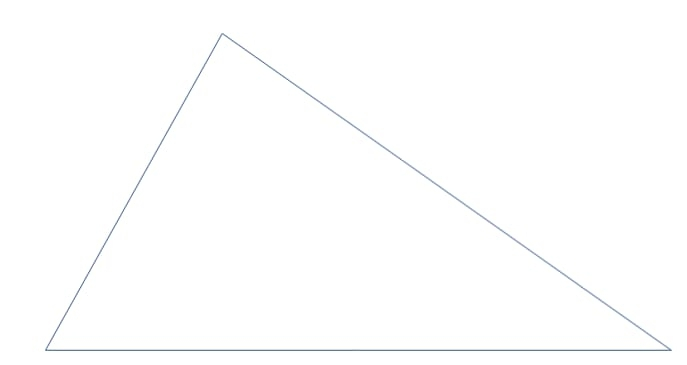Teacher guides pupils to measure and record each length to find the perimeter.

ACTIVITY 3 – WORKING EXERCISE

Measure and find the perimeter,### LESSON 3 – PERIMETER OF SQUARES AND RECTANGLES

ACTIVITY 1 – SQUARE AND RECTANGLE

Square and rectangle are any shape with four lengths.

Square has 4 equal length while rectangle has 2 equal opposite lengths.

ACTIVITY 2 – PERIMETER OF SQUARES

Find the perimeter of,Perimeter is 5 cm + 5 cm + 5 cm + 5 cm = 25 cm.

ACTIVITY 3 – PERIMETER OF RECTANGLES

Find the perimeter of,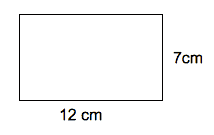Perimeter is 7 cm + 12 cm + 7 cm + 12 cm = 38 cm.

ACTIVITY 4 – PERIMETER OF SQUARES AND RECTANGLES

Teacher guides pupils to measure, record and find the perimeter of the following:

###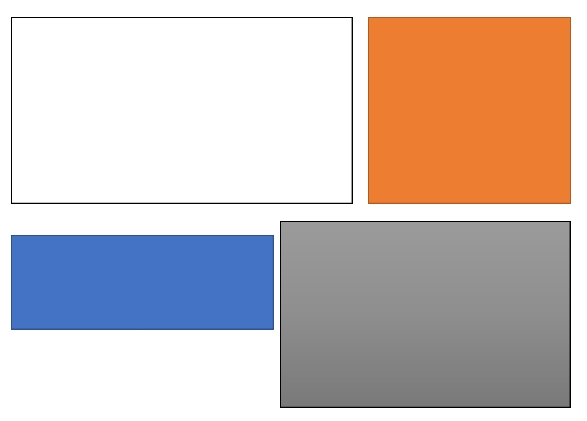ACTIVITY 5 – WORKING EXERCISE

Find the perimeter of the following: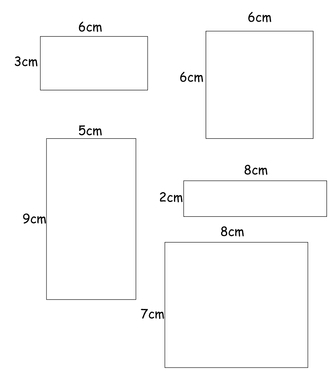### LESSON 4 – OUTDOOR ACTIVITIES

Teacher guides pupils out to measure, record and find the perimeter of the school’s building using non standard and standard tools.

LESSON 5 – REVISION AND WEEKLY ASSESSMENT

As stated in performance objectives or lesson evaluation.

### PRESENTATION

To deliver the lesson, the teacher adopts the following steps:

1. To introduce the lesson, the teacher revises the previous lesson. Based on this, he/she asks the pupils some questions;

2. Teacher organizes pupils in groups or pairs depending on the size of the class.

3. Teacher guides pupils to count from 861 – 880 and skip count in 3s, 5s, 6s, 9s and 10s.

Pupil’s Activities – Count from 861 – 880 and skip count in 3s, 5s, 6s, 9s and 10s.

4. Teacher reviews the meaning and properties of triangles, squares and rectangles.

Pupil’s Activities – State the properties of triangles, squares and rectangles.

5. Teacher uses pupils active participation to introduce the perimeter of a triangle, square and rectangle.

Pupil’s Activities – Pay attention to the lesson introduction.

6. Teacher guides pupils to find the perimeter of a  triangle, square and rectangle with specific lengths.

7. Teacher also guides pupils to measure, record and find the perimeter of a triangle, square and rectangle.

Pupil’s Activities – Find the perimeter of a triangle, square and rectangle.

8. Teacher guides pupils to determine the shapes of some building within the school and also to measure, record and find the a building using standard and non standard measuring tools.

Pupil’s Activities – Measure, record and find the a building using standard and non standard measuring tools.

9. Summarizes the lessons on the board on the board with appropriate lesson evaluation.

Pupil’s Activities – Participate actively in the summary of the lesson by responding correctly as the questions and write as instructed.

### CONCLUSION

To conclude the lesson for the week, the teacher revises the entire lesson and links it to the following week’s lesson.

### NEXT LESSON

Introduction to Area of Squares and Rectangles by Counting and Using Formula | Counting of Numbers from 881 – 900 (Test) Primary 3 (Basic 3) Term 3 Week 4 Mathematics

### LESSON EVALUATION

1. count from 861 – 880.

2. skip count in 3, 5, 7s, 9s and 10s.

3. find the perimeter of triangles, squares and rectangles.

### ATTEMPT ALL THE QUESTIONS

1. _________ is the distance around a particular shape.

A. Square

B. Rectangle

C. Perimeter

Figure 1.0 Square and Rectangular shapes.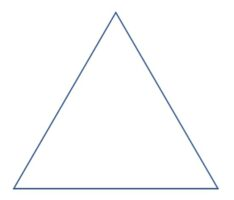Use the figure 1.0 to answer questions 2 – 5.

2. Measure and record the lengths of the triangle.

3. Find the perimeter of the triangular shape.

4. Measure and record the lengths of the square.

5. Find the of the square shape.

6. Measure and record the lengths of the rectangle.

7. Find the perimeter of the rectangular shape.

8. Write numbers from 861 – 880.

9. Skip count in 3s, 6s, and 7s from 861 – 880.

10. Skip count in 9s and 10s from 800 to 880